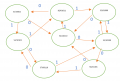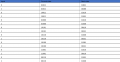# 8 state diagram to digital circuits

#### rpx89

Joined Nov 13, 2022
6
Hi there,

I have an exercise at school in which I have to come up with a state table for a state diagram. Here is what I have done so farNow from this, I need to come up with the circuits but I don't know how to do a Karnaugh map for these?
There is one input as you can see and then 5 variables (ie. A,B,C,D,E). I intend to use D flip flops.
It would be greatly appreciated if someone could explain or give an example of let's say the karnaugh map for variable A, just so I understand how to proceed from there?

Thank you so much

#### WBahn

Joined Mar 31, 2012
27,934
Why are you using five bits for your state designator when you only need three (for eight states)? Are those five bit value the output when in a particular state?

#### rpx89

Joined Nov 13, 2022
6
Why are you using five bits for your state designator when you only need three (for eight states)? Are those five bit value the output when in a particular state?
This is why I was asking as I am not too sure this table is correct. I have used 5 bits as these are purely the current state and next state when input is at 0 or 1 if you look at the state diagram. For example, for S0, current state is 01011 and then if input is 0 next state is 10011. I am not sure how else to create the state table for 5 variables.

#### WBahn

Joined Mar 31, 2012
27,934
This is why I was asking as I am not too sure this table is correct. I have used 5 bits as these are purely the current state and next state when input is at 0 or 1 if you look at the state diagram. For example, for S0, current state is 01011 and then if input is 0 next state is 10011. I am not sure how else to create the state table for 5 variables.
But why are you using five variables in the first place? You only have eight states, so you only need three variables to establish the state. You then have one input variable, so your K-maps will be four variables, which is a lot easier for humans to work with than a six-variable K-map.

I think you might be confusing the notion of "state" with the notion of "output". When you are in S0, do you have five outputs that are supposed to be 01011 whenever you are in S0?

#### rpx89

Joined Nov 13, 2022
6
But why are you using five variables in the first place? You only have eight states, so you only need three variables to establish the state. You then have one input variable, so your K-maps will be four variables, which is a lot easier for humans to work with than a six-variable K-map.

I think you might be confusing the notion of "state" with the notion of "output". When you are in S0, do you have five outputs that are supposed to be 01011 whenever you are in S0?
Yes I think I am confusing both. So would you be able to help me by confirming, is the S0/01011 just a state variable name where the actual state is 000?

Would you be able to give me an example for example of how a first line of my state table would look like?

Thank you very much for your kind help so far!

#### WBahn

Joined Mar 31, 2012
27,934
Yes I think I am confusing both. So would you be able to help me by confirming, is the S0/01011 just a state variable name where the actual state is 000?

Would you be able to give me an example for example of how a first line of my state table would look like?

Thank you very much for your kind help so far!
You can assign any code (of at least three bits) to represent each state. However, convention is that the number of the state, so 0 for S0 and 7 for S7 and so forth, is the code that is used. But at the level of your state transition table, this can be abstracted away by just using the state labels directly.

The tradition format for a state transition table is to list the states in the first column and then the possible control/input values as the subsequent columns. In each cell is then the state that the machine will transition to next.

Code:
     Input
Sn   0   1
S0  S1  S2
You have a couple of options in doing your design. First, you can just use the state labels to base your state codes on and then plunge ahead. An alternative is to spend time looking at whether there is a more elegant choice of codes to use that will simplify the resulting logic. While this is often the case, it is not guaranteed (nor is it guaranteed that you will find it even if one does exist) and you can spend a considerable amount of time looking for it. Also, keep in mind that you don't have to use just three bits for your state codes. If you want to, you certainly can use your five outputs as your state codes (provided they are all distinct, which they are in your case).

This is part of the art of engineering -- choosing from among several available approaches, each of which usually comes with a set of pros and cons.